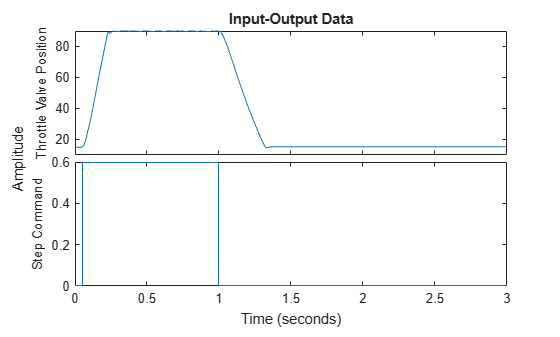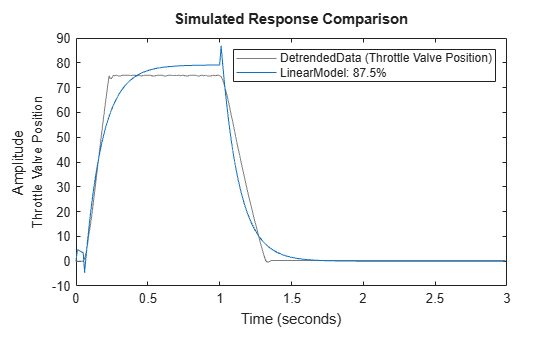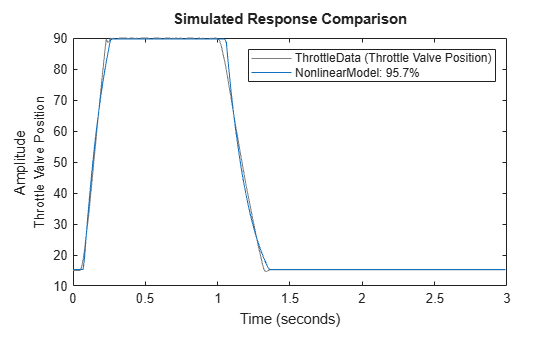# Estimate Hammerstein-Wiener Models Initialized Using Linear OE Models

This example shows how to estimate Hammerstein-Wiener models using linear OE models.

`load throttledata.mat`

This command loads the data object `ThrottleData` into the workspace. The object contains input and output samples collected from an engine throttle system, sampled at a rate of 100Hz.

A DC motor controls the opening angle of the butterfly valve in the throttle system. A step signal (in volts) drives the DC motor. The output is the angular position (in degrees) of the valve.

Plot the data to view and analyze the data characteristics.

`plot(ThrottleData)`In the normal operating range of 15-90 degrees, the input and output variables have a linear relationship. You use a linear model of low order to model this relationship.

In the throttle system, a hard stop limits the valve position to `90` degrees, and a spring brings the valve to `15` degrees when the DC motor is turned off. These physical components introduce nonlinearities that a linear model cannot capture.

Estimate a Hammerstein-Wiener model to model the linear behavior of this single-input single-output system in the normal operating range.

```% Detrend the data because linear models cannot capture offsets. Tr = getTrend(ThrottleData); Tr.OutputOffset = 15; DetrendedData = detrend(ThrottleData,Tr); % Estimate a linear OE model with na=2, nb=1, nk=1. opt = oeOptions('Focus','simulation'); LinearModel = oe(DetrendedData,[2 1 1],opt);```

Compare the simulated model response with estimation data.

`compare(DetrendedData, LinearModel)`The linear model captures the rising and settling behavior in the linear operating range but does not account for output saturation at 90 degrees.

Estimate a Hammerstein-Wiener model to model the output saturation.

`NonlinearModel = nlhw(ThrottleData, LinearModel, [], 'saturation');`

The software uses the orders and delay of the linear model for the orders of the nonlinear model. In addition, the software uses the B and F polynomials of the linear transfer function.

Compare the nonlinear model with data.

`compare(ThrottleData, NonlinearModel)`## Related Topics

##### Support